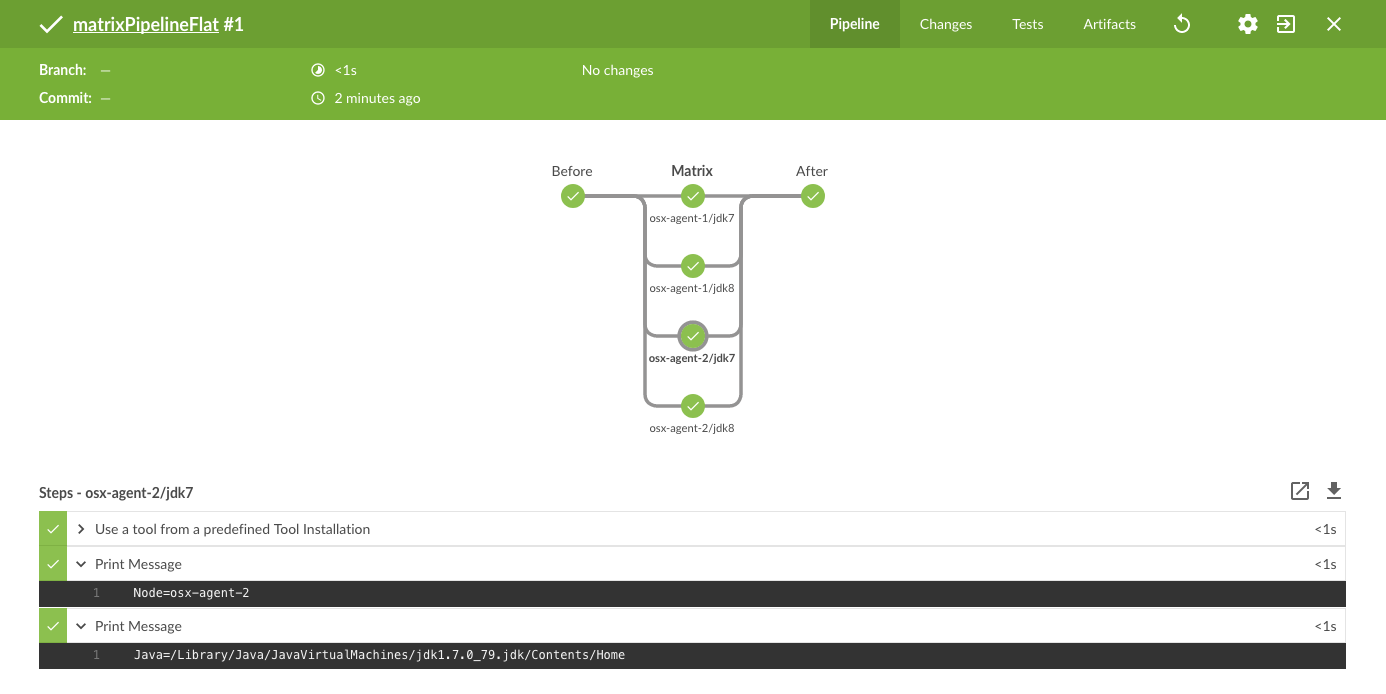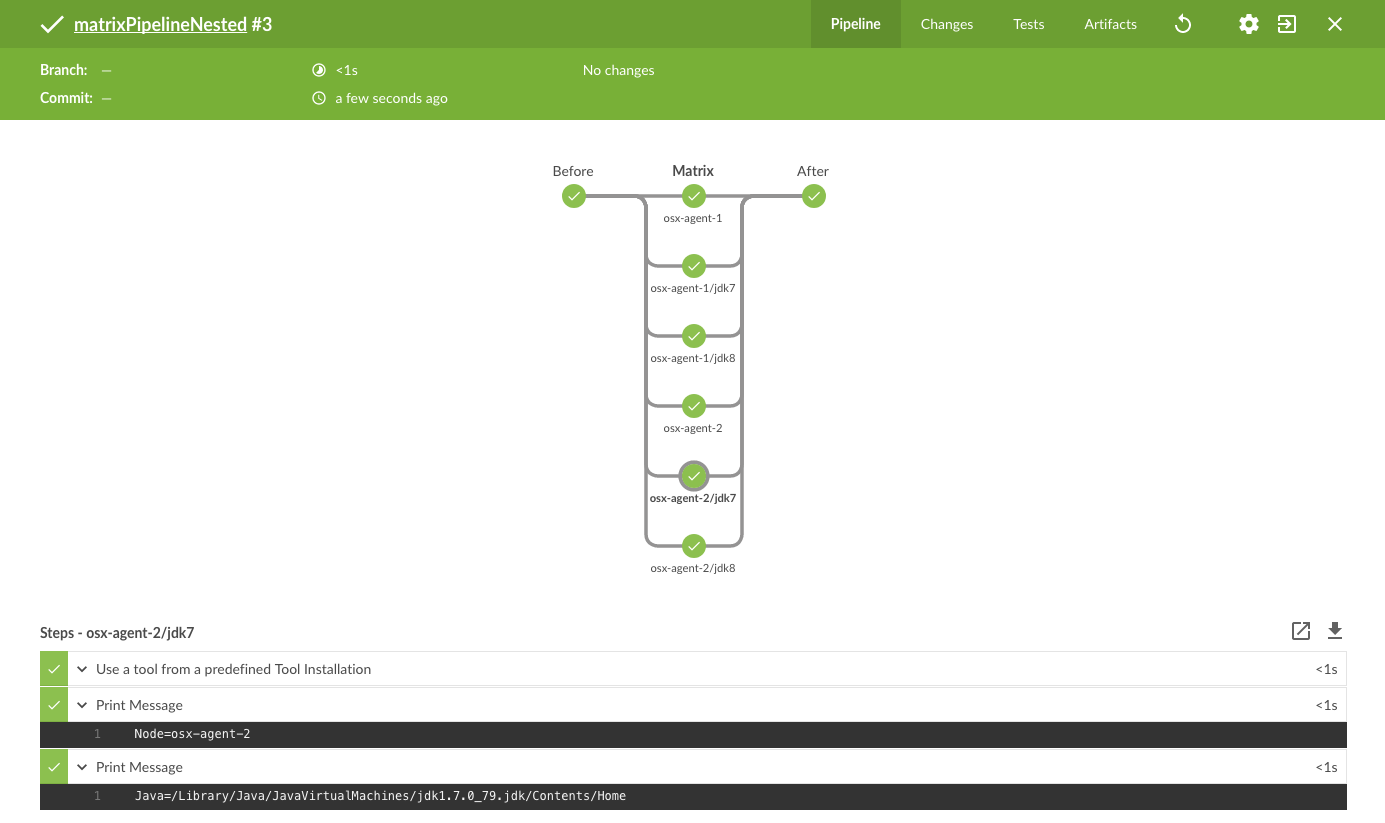# Create a Matrix-like flow with Pipeline

Article ID:115000088431

## Issue

• I would like to create a Pipeline that runs parallel task in the same way a Matrix job does

## Resolution

You can run a pipeline that mocks the behavior of a Matrix jobs. You can trivially run the different combinations in parallel and express the structure like a Matrix.

In terms of Visualization however, Pipeline Stage View is not able to display the result in a Matrix structure. There are long standing improvement issues about this: JENKINS-27395 and JENKINS-33185.

Blue Ocean Plugin offer better visualization capabilities and is the way forward.

#### Example with "Flat" structure:

The following pipeline has 2 axes: Nodes and JDKs. Therefore there should be 4 tasks running in parallel:

• osx-agent-1 / jdk7: Runs on node "osx-agent-1" and use the tool "jdk7"

• osx-agent-1 / jdk8: Runs on node "osx-agent-1" and use the tool "jdk8"

• osx-agent-2 / jdk7: Runs on node "osx-agent-2" and use the tool "jdk7"

• osx-agent-2 / jdk8: Runs on node "osx-agent-2" and use the tool "jdk8"

```def axisNode = ["osx-agent-1","osx-agent-2"]
def axisTool = ["jdk7","jdk8"]

stage("Before") {
node {
echo "before"
}
}

for(int i=0; i< axisNode.size(); i++) {
def axisNodeValue = axisNode[i]
for(int j=0; j< axisTool.size(); j++) {
def axisToolValue = axisTool[j]
node(axisNodeValue) {
def javaHome = tool axisToolValue
println "Node=\${env.NODE_NAME}"
println "Java=\${javaHome}"
}
}
}
}

stage ("Matrix") {
}

stage("After") {
node {
echo "after"
}
}```

Note that the "Matrix stage" is a simple parallel task and the matrix structure can be part of a bigger pipeline (as shown by this pipeline that is creating stages before and after the matrix structure).

Visualization in Blue Ocean#### Example with "Nested" structure:

Another example is to run Nested Parallel tasks. The following pipeline do the exact same things except that we create a nest parallel tasks:

• "osx-agent-1": Parent stage

• osx-agent-1 / jdk7: Runs on node "osx-agent-1" and use the tool "jdk7"

• osx-agent-1 / jdk8: Runs on node "osx-agent-1" and use the tool "jdk8"

• "osx-agent-2": Parent stage

• osx-agent-2 / jdk7: Runs on node "osx-agent-2" and use the tool "jdk7"

• osx-agent-2 / jdk8: Runs on node "osx-agent-2" and use the tool "jdk8"

```def axisNode = ["osx-agent-1","osx-agent-2"]
def axisTool = ["jdk7","jdk8"]

stage("Before") {
node {
echo "before"
}
}

for(int i=0; i< axisNode.size(); i++) {
def axisNodeValue = axisNode[i]
for(int j=0; j< axisTool.size(); j++) {
def axisToolValue = axisTool[j]
def javaHome = tool axisToolValue
println "Node=\${env.NODE_NAME}"
println "Java=\${javaHome}"
}
}
node(axisNodeValue) {
}
}
}

stage ("Matrix") {